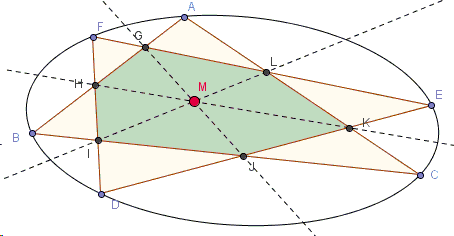# Two Triangles Inscribed in a Conic - with Elementary Solution

### Jack D'Aurizio

The following ideas come from Gabriel Giorgieri, and I am grateful to him. (The theorem below constitutes the statement suggested by Vladimir Nikolin and generalized by Hubert Shutrick.)

Assume that two triangles $ABC$ and $DEF$ are inscribed in the same conic and intersect in six points $G,H,I,J,K,L.$Then the lines $GJ$, $HK$, $IL$ are concurrent.

(The applet below illustrates the proof. Points $A$, $B$, $C$, $D$, $E$, $F$ are draggable.)

### Proof

Without loss of generality, we can assume that the circumscribed conic is a circle. If we call $X$ the intersection of $CF$ and $BE,$ $Y$ the intersection of $AD$ and $CF,$ $Z$ the intersection of $AD$ and $BE,$ by the Pascal theorem we know that $G,$ $Y,$ $J$ are aligned, and the same holds for $H,$ $X,$ $K,$ and $I,$ $Z,$ $L.$ So we only need to prove that $GY,$ $HX,$ $IZ$ are concurrent cevians with respect to the triangle $XYZ$.In order to apply the Trig Ceva theorem, we express the ratio $\displaystyle\frac{\mbox{sin}(ZXK)}{\mbox{sin}(KXY)}$ by exploiting the fact that in $\Delta BFX$ the cevians $KX,$ $AB,$ $DF$ concur in $H.$ What we get is:

\displaystyle \begin{align} \frac{\mbox{sin}(ZXK)}{\mbox{sin}(KXY)} &= \frac{\mbox{sin}(HXB)}{\mbox{sin}(HXF)} \\ &= \frac{\mbox{sin}(BFH)}{\mbox{sin}(HFX)} \cdot \frac{\mbox{sin}(HBX)}{\mbox{sin}(HBF)}, \end{align}

so, by denoting with $[UV]$ the sine of half the angle between $U$ and $V$ in the circumcircle of $\Delta ABC,$ we get:

$\displaystyle \frac{\mbox{sin}(ZXK)}{\mbox{sin}(KXY)} = \frac{[BD]}{[CD]} \cdot \frac{[AE]}{[AF]},$

and, in the same way:

$\displaystyle\frac{\mbox{sin}(XYG)}{\mbox{sin}(GYZ)} = \frac{[CE]}{[AE]} \cdot \frac{[BF]}{[BD]}, \\ \displaystyle\frac{\mbox{sin}(YZI)}{\mbox{sin}(IZX)} = \frac{[AF]}{[BF]}\cdot \frac{[CD]}{[CE]}.$

The product of the last three right-hand sides is one, proving that the lines $XK,$ $YG,$ $ZI$ are concurrent, QED.

As already noted, this concurrence of Pascal lines is different from the ones stated by Steiner and Kirkman. This theorem should have a proper name! :)69724825